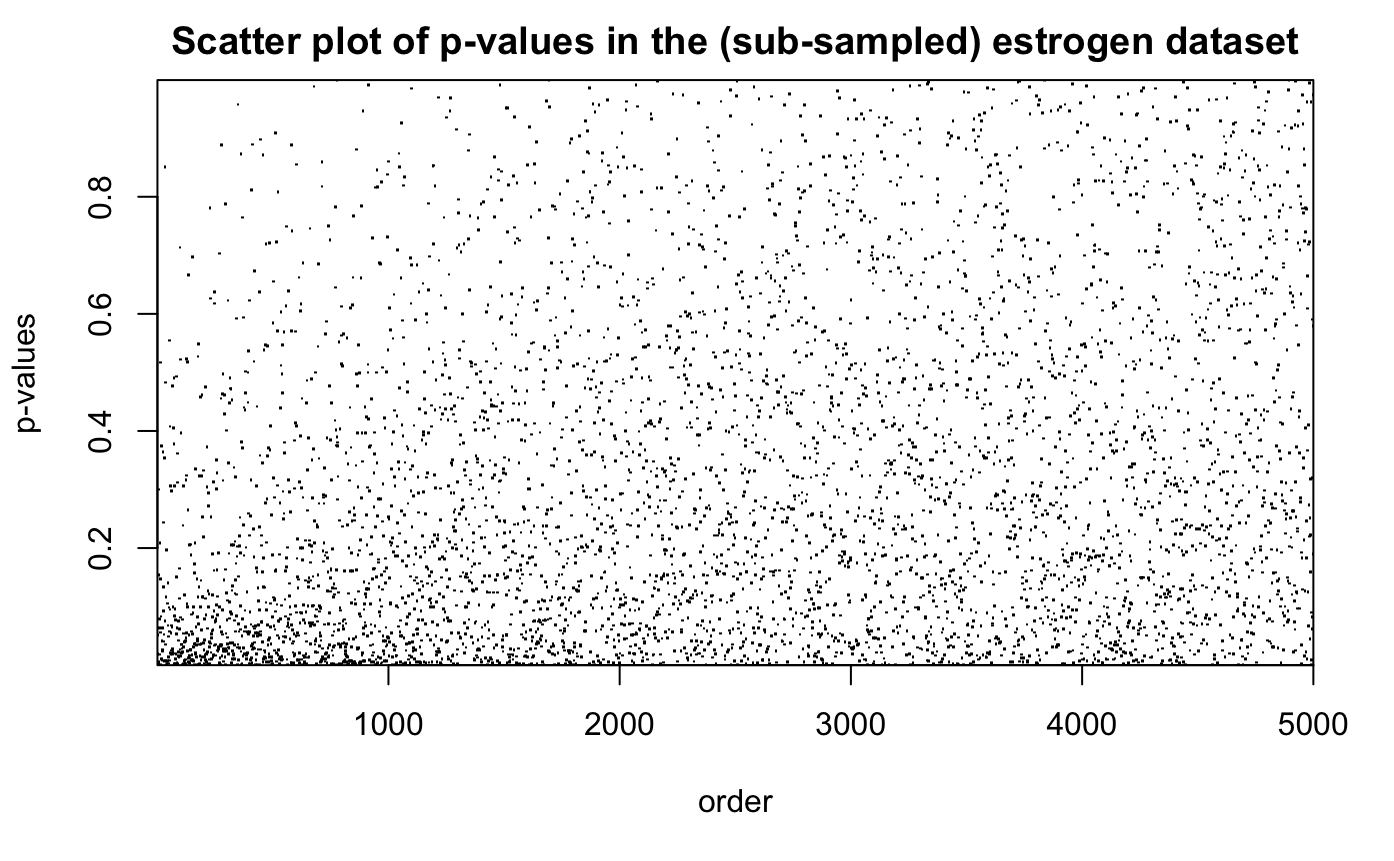This is a demo of our adaptMT package. adaptMT has two main components: an API that allows users to specify the working model and algorithms to fit them, as well as a pool of easy-to-use end-to-end wrappers. The former is captured by function adapt. The latter includes adapt_glm, adapt_gam and adapt_glmnet in current version (0.1.3.9000) for using GLM, GAM and L1-regularized GLM.

# install the "adaptMT" package from github.
# will be submitted to CRAN very soon.
devtools::install_github("lihualei71/adaptMT")
library("adaptMT")

We illustrate one of the main function adapt_glm, for AdaPT with logistic-Gamma GLM as the working model, on estrogen dataset, a gene/drug response dataset from NCBI Gene Expression Omnibus (GEO). estrogen dataset consists of gene expression measurements for $$n = 22283$$ genes, in response to estrogen treatments in breast cancer cells for five groups of patients, with different dosage levels and 5 trials in each. The task is to identify the genes responding to a low dosage. The p-values pi for gene i is obtained by a one-sided permutation test which evaluates evidence for a change in gene expression level between the control group (placebo) and the low-dose group. $$\{p_i : i \in [n]\}$$ are then ordered according to permutation t-statistics comparing the control and low-dose data, pooled, against data from a higher dosage (with genes that appear to have a strong response at higher dosages placed earlier in the list). The code to compute p-values and the ordering can be found in Rina Barber’s website.

In this demo, we subsample the top 5000 genes for illustration.

# load the data.
data("estrogen")
# Take the first 5000 genes
library("dplyr")
estrogen <- select(estrogen, pvals, ord) %>%
filter(ord <= 5000)
rownames(estrogen) <- NULL
head(estrogen, 5)
summary(estrogen)
     pvals               ord
Min.   :0.000011   Min.   :   1
1st Qu.:0.076082   1st Qu.:1251
Median :0.238279   Median :2500
Mean   :0.315094   Mean   :2500
3rd Qu.:0.501009   3rd Qu.:3750
Max.   :0.999289   Max.   :5000Now we execute adapt_glm on this dataset. adapt_glm assumes a conditional logistic-Gamma GLM as the working model. Specifically, it models the p-values as $H_i \mid x_i \sim \pi(x_i), \quad \mathrm{logit}(\pi(x_i))= \phi(x_i)^{T}\beta$ $-\log p_i \mid H_i, x_i\sim \left\{\begin{array}{ll} \mathrm{Exp(1)} & H_i = 0\\ \mathrm{Exp(\mu(x))} & H_i = 1\end{array}\right., \quad \frac{1}{\mu(x_i)} = \phi(x_i)^{T}\gamma$ where $$\phi(x)$$ is a featurization of $$x$$. In this example, we use the spline bases, given by ns function from splines package. For illustration, we choose our candidate models as the above GLMs with $$\phi(x)$$ being the spline bases with equal-spaced knots and the number of knots ranging from 6-10. We use BIC to select the best model at the initial stage and use the selected model for the following model fitting.

# prepare the inputs of AdaPT
# need "splines" package to construct the formula for glm
library("splines")
pvals <- as.numeric(estrogen$pvals) x <- data.frame(x = as.numeric(estrogen$ord))
formulas <- paste0("ns(x, df = ", 6:10, ")")
formulas
 "ns(x, df = 6)"  "ns(x, df = 7)"  "ns(x, df = 8)"  "ns(x, df = 9)"  "ns(x, df = 10)"

adapt_glm function provides several user-friendly tools to monitor the progress. For model selection, a progress bar will, by default, be shown in the console that indicates how much proportion of models have been fitted. This can be turned off by setting verbose$ms = FALSE. Similarly for model fitting, a progress bar can be shown in the console, though not by default, by setting verbose$fit = TRUE. Also, by default, the progress of the main process will be shown in the console that indicates (1) which target FDR level has been achieved; (2) FDPhat for each target FDR level; (3) number of rejections for each target FDR level.

# run AdaPT
res <- adapt_glm(x = x, pvals = pvals, pi_formulas = formulas, mu_formulas = formulas)
Model selection starts!
Shrink the set of candidate models if it is too time-consuming.

|
|                                                  |   0%
|
|==========                                        |  20%
|
|====================                              |  40%
|
|==============================                    |  60%
|
|========================================          |  80%
|
|==================================================| 100%
alpha = 0.29: FDPhat 0.2899, Number of Rej. 3450
alpha = 0.28: FDPhat 0.28, Number of Rej. 3347
alpha = 0.27: FDPhat 0.2699, Number of Rej. 3227
alpha = 0.26: FDPhat 0.26, Number of Rej. 3104
alpha = 0.25: FDPhat 0.2498, Number of Rej. 3022
alpha = 0.24: FDPhat 0.2397, Number of Rej. 2937
alpha = 0.23: FDPhat 0.2297, Number of Rej. 2891
alpha = 0.22: FDPhat 0.2199, Number of Rej. 2760
alpha = 0.21: FDPhat 0.21, Number of Rej. 2700
alpha = 0.2: FDPhat 0.1999, Number of Rej. 2586
alpha = 0.19: FDPhat 0.1899, Number of Rej. 2501
alpha = 0.18: FDPhat 0.1798, Number of Rej. 2381
alpha = 0.17: FDPhat 0.1699, Number of Rej. 2272
alpha = 0.16: FDPhat 0.1598, Number of Rej. 2165
alpha = 0.15: FDPhat 0.1497, Number of Rej. 2064
alpha = 0.14: FDPhat 0.1397, Number of Rej. 1954
alpha = 0.13: FDPhat 0.1297, Number of Rej. 1889
alpha = 0.12: FDPhat 0.1196, Number of Rej. 1781
alpha = 0.11: FDPhat 0.1099, Number of Rej. 1720
alpha = 0.1: FDPhat 0.0996, Number of Rej. 1586
alpha = 0.09: FDPhat 0.0897, Number of Rej. 1405
alpha = 0.08: FDPhat 0.0798, Number of Rej. 1241
alpha = 0.07: FDPhat 0.0698, Number of Rej. 1074
alpha = 0.06: FDPhat 0.0598, Number of Rej. 937
alpha = 0.05: FDPhat 0.0498, Number of Rej. 884
alpha = 0.04: FDPhat 0.0399, Number of Rej. 777
alpha = 0.03: FDPhat 0.0289, Number of Rej. 519
alpha = 0.02: FDPhat 0.0179, Number of Rej. 224

plot_thresh_1d gives the plot for the rejection threshold as a function of x (must be univariate without repeated value) for given $$\alpha$$. We display the plots for $$\alpha \in \{0.3, 0.25, 0.2, 0.15, 0.1, 0.05\}$$.